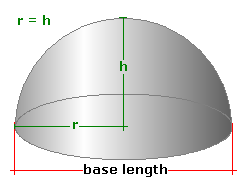Spike's Calculators

# Hemisphere Dome Tank - FeetCalculate the volume of a dome-shaped tank/container that has the shape of a hemisphere (half sphere).

Half of the length's base equals the height in this type of tank. This calculator uses feet for measurements.

##### Approximate weight of Fuel or Water
```one US gallon of water = 8.34 pounds.
one US gallon of diesel fuel = 7.1 pounds
one US gallon of gas = 6 pounds
```

### Hemispherical Dome Tank

Tank Diameter ft in
Liquid Weight-Pounds per US Gallon lb/gal US

#### Results:

###### Tank Volume
In Cubic Feet ft³
In Cubic Inches in³
Cubic Metres
###### Tank Capacity
In US Gallons gal US
In Imperial Gallons gal Imperial
In Litres L
###### Liquid Weight and Surface Area
Weight of Liquid lbs
Surface Area in Square Feet ft²
In Square Inches in²
In Square Millimetres mm²
In Square Metres

#### Calculator

1. enter the tank's base length in feet
2. the weight of the liquid in pounds per US gallon

#### Results

1. the volume of the tank in cubic feet
2. volume in cubic inches
3. volume in cubic metres
4. tank capacity in US gallons
5. capacity in Imperial gallons
6. capacity in litres
7. weight of the liquid when the tank is full
8. the surface area of the tank in square feet
9. the surface area in square inches
10. the surface area in square millimetres
11. the surface area in square metres

##### Formula
```V = 2/3Πr³
where V is the volume
Π = 3.14159265
r half the length of the tank base
```
##### Conversions
```one cubic foot (ft³) = 1728 cubic inches (in³)
one cubic foot (ft³) = 28316846.592 cubic millimetres (mm³)
one cubic foot (ft³) = 0.028316846592 cubic metres (m³)
one cubic foot (ft³) = 7.48051948051947 gallons (gal) US
one cubic foot (ft³) = 6.22883545904283 gallons (gal) Imperial
one square foot (ft²) = 144 square inches (in²)
one square foot (ft²) = 92903.04 square millimetres (mm²)
one square foot (ft²) = 0.09290304 square metres (m²)
```

### Hemispherical Shaped Dome Tank/Container (Half Sphere)#### Filled